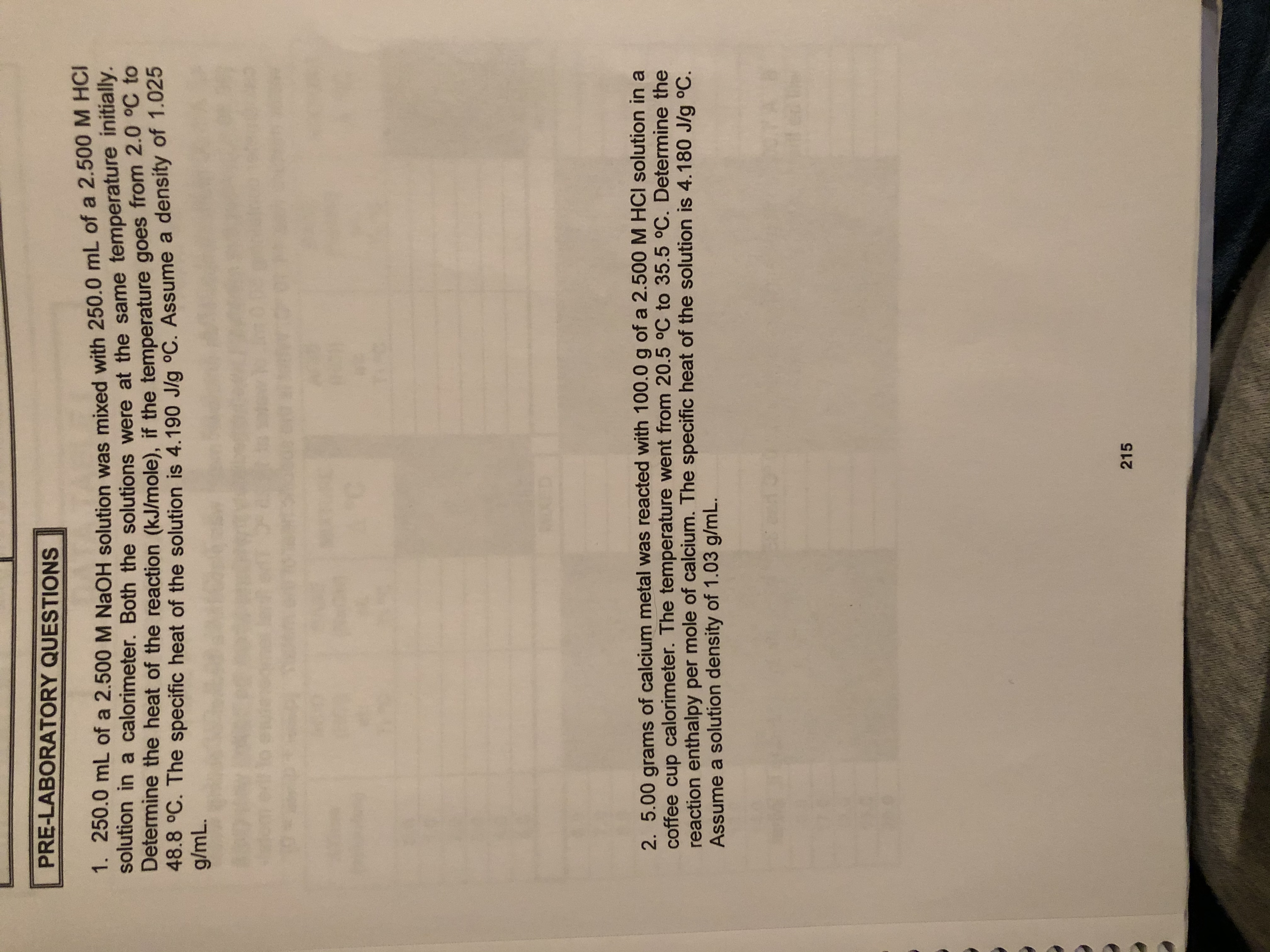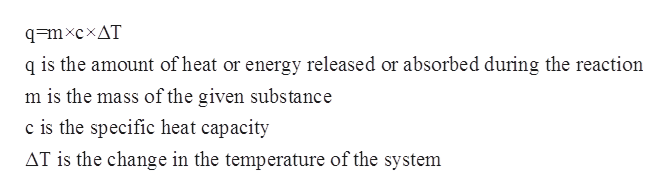# PRE-LABORATORY QUESTIONS1. 250.0 mL of a 2.500 M NaOH solution was mixed with 250.0 mL of a 2.500 M HCIltion in a calorimeter. Both the solutions were at the same temperature initially.Determine the heat of the reaction (kJ/mole), if the temperature goes from 2.0 °C to48.8 °C. The specific heat of the solution is 4.190 Jlg °C. Assume a density of 1.025g/mL.KETD2. 5.00 grams of calcium metal was reacted with 100.0 g of a 2.500 M HCI solution in acoffee cup calorimeter. The temperature went from 20.5 °C to 35.5 °C. Determine thereaction enthalpy per mole of calcium. The specific heat of the solution is 4.180 J/g oC.Assume a solution density of 1.03 g/mL.215

Question
3 views

Question 1help_outlineImage TranscriptionclosePRE-LABORATORY QUESTIONS 1. 250.0 mL of a 2.500 M NaOH solution was mixed with 250.0 mL of a 2.500 M HCI ltion in a calorimeter. Both the solutions were at the same temperature initially. Determine the heat of the reaction (kJ/mole), if the temperature goes from 2.0 °C to 48.8 °C. The specific heat of the solution is 4.190 Jlg °C. Assume a density of 1.025 g/mL. KET D 2. 5.00 grams of calcium metal was reacted with 100.0 g of a 2.500 M HCI solution in a coffee cup calorimeter. The temperature went from 20.5 °C to 35.5 °C. Determine the reaction enthalpy per mole of calcium. The specific heat of the solution is 4.180 J/g oC. Assume a solution density of 1.03 g/mL. 215 fullscreen
check_circle

Step 1

The specific heat capacity for a given chemical substance or a solution can be defined as the amount of heat which is required to raise the temperature of a given substance per unit of its mass. The mathematical relationship between specific heat capacity, mass, change in temperature and the heat of the solution is given by the following equation:help_outlineImage Transcriptioncloseq=mxcxAT q is the amount of heat or energy released or absorbed during the reaction m is the mass of the given substance c is the specific heat capacity AT is the change in the temperature of the system fullscreen
Step 2

The initial temperature is given to be 2.0oC

The final temperature after is given to be 48.8 oC

The specific heat capacity of solution is given to be 4.190 J g-1 oC-1.

The density of the solution is given to be 1.025 g/mL.

The molarity and the volume of the NaOH and HCl solution is given to be 2.500 M and 250 mL respectively.

Hence, the total volume of the mixture will be 250 mL+250 mL=500 mL.

The mass of the solution can be calculated as follows:

Step 3

The heat which is absorbed or released during th...

### Want to see the full answer?

See Solution

#### Want to see this answer and more?

Solutions are written by subject experts who are available 24/7. Questions are typically answered within 1 hour.*

See Solution
*Response times may vary by subject and question.
Tagged in

### Chemistry